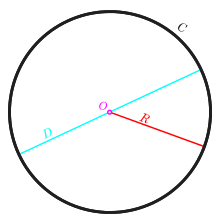For other uses, see Radius (disambiguation).Circle with circumference C in black, diameter D in cyan, radius R in red, and centre or origin O in magenta.

In classical geometry, a radius of a circle or sphere is any of the line segments from its center to its perimeter, and in more modern usage, it is the length of any of them. The name comes from Latin radius, meaning "ray" but also the spoke of a chariot wheel. The plural of radius can be either radii (from the Latin plural) or the conventional English plural radiuses. The typical abbreviation and mathematic variable name for "radius" is r. By extension, the diameter d is defined as twice the radius:If an object does not have a center, the term may refer to its circumradius, the radius of its circumscribed circle or circumscribed sphere. In either case, the radius may be more than half the diameter, which is usually defined as the maximum distance between any two points of the figure. The inradius of a geometric figure is usually the radius of the largest circle or sphere contained in it. The inner radius of a ring, tube or other hollow object is the radius of its cavity.

For regular polygons, the radius is the same as its circumradius. The inradius of a regular polygon is also called apothem. In graph theory, the radius of a graph is the minimum over all vertices u of the maximum distance from u to any other vertex of the graph.

The radius of the circle with perimeter (circumference) C isAlternatively, this can be expressed aswith(tau) being equal toexactly, although this has yet to gain mainstream usage.

## Formulae

For many geometrical figures, the radius has a well-defined relationship with other measures of the figure.

### Circles

The radius of a circle with area A isThe radius of a circle that is connecting the three points P1, P2 and P3 is given bywhere θ is the angleThis formula uses the sine rule. If the three points are given by their coordinates,and, the radius can be expressed as### Regular polygons

The radius of a regular polygon with n sides of length s is given by, with### Hypercubes

The radius of a d-dimensional hypercube with side s is## Use in coordinate systems

### Polar coordinates

The polar coordinate system is a two-dimensional coordinate system in which each point on a plane is determined by a distance from a fixed point and an angle from a fixed direction.

The fixed point (analogous to the origin of a Cartesian system) is called the pole, and the ray from the pole in the fixed direction is the polar axis. The distance from the pole is called the radial coordinate or radius, and the angle is the angular coordinate, polar angle, or azimuth.

### Cylindrical coordinates

In the cylindrical coordinate system, there is a chosen reference axis and a chosen reference plane perpendicular to that axis. The origin of the system is the point where all three coordinates can be given as zero. This is the intersection between the reference plane and the axis.

The axis is variously called the cylindrical or longitudinal axis, to differentiate it from the polar axis, which is the ray that lies in the reference plane, starting at the origin and pointing in the reference direction.

The distance from the axis may be called the radial distance or radius, while the angular coordinate is sometimes referred to as the angular position or as the azimuth. The radius and the azimuth are together called the polar coordinates, as they correspond to a two-dimensional polar coordinate system in the plane through the point, parallel to the reference plane. The third coordinate may be called the height or altitude (if the reference plane is considered horizontal), longitudinal position, or axial position.

### Spherical coordinates

In a spherical coordinate system, the radius describes the distance of a point from a fixed origin. Its position if further defined by the polar angle measured between the radial direction and a fixed zenith direction, and the azimuth angle, that is, the angle between the orthogonal projection of the radial direction on a reference plane that passes through the origin and is orthogonal to the zenith, and a fixed reference direction in that plane.

## References

1. Definition of Radius at dictionary.reference.com. Accessed on 2009-08-08.
2. "Radius - Definition and More from the Free Merriam-Webster Dictionary". Merriam-webster.com. Retrieved 2012-05-22.
3. Definition of radius at mathwords.com. Accessed on 2009-08-08.
4. Barnett Rich, Christopher Thomas (2008), Schaum's Outline of Geometry, 4th edition, 326 pages. McGraw-Hill Professional. ISBN 0-07-154412-7, ISBN 978-0-07-154412-2. Online version accessed on 2009-08-08.
5. Jonathan L. Gross, Jay Yellen (2006), Graph theory and its applications. 2nd edition, 779 pages; CRC Press. ISBN 1-58488-505-X, 9781584885054. Online version accessed on 2009-08-08.
6. Hartl, Michael. The Tau Manifesto. http://www.tauday.com/tau-manifesto
7. Brown, Richard G. (1997). Andrew M. Gleason, ed. Advanced Mathematics: Precalculus with Discrete Mathematics and Data Analysis. Evanston, Illinois: McDougal Littell. ISBN 0-395-77114-5.
8. Krafft, C.; Volokitin, A. S. (1 January 2002). "Resonant electron beam interaction with several lower hybrid waves". Physics of Plasmas. 9 (6): 2786–2797. Bibcode:2002PhPl....9.2786K. doi:10.1063/1.1465420. ISSN 1089-7674. Retrieved 9 February 2013. ...in cylindrical coordinates (r,θ,z) ... and Z=vbzt is the longitudinal position...
9. Alexander Groisman and Victor Steinberg (1997), Solitary Vortex Pairs in Viscoelastic Couette Flow. Physical Review Letters, volume 78, number 8, 1460–1463. doi:10.1103/PhysRevLett.78.1460 "[...]where r, θ, and z are cylindrical coordinates [...] as a function of axial position[...]"